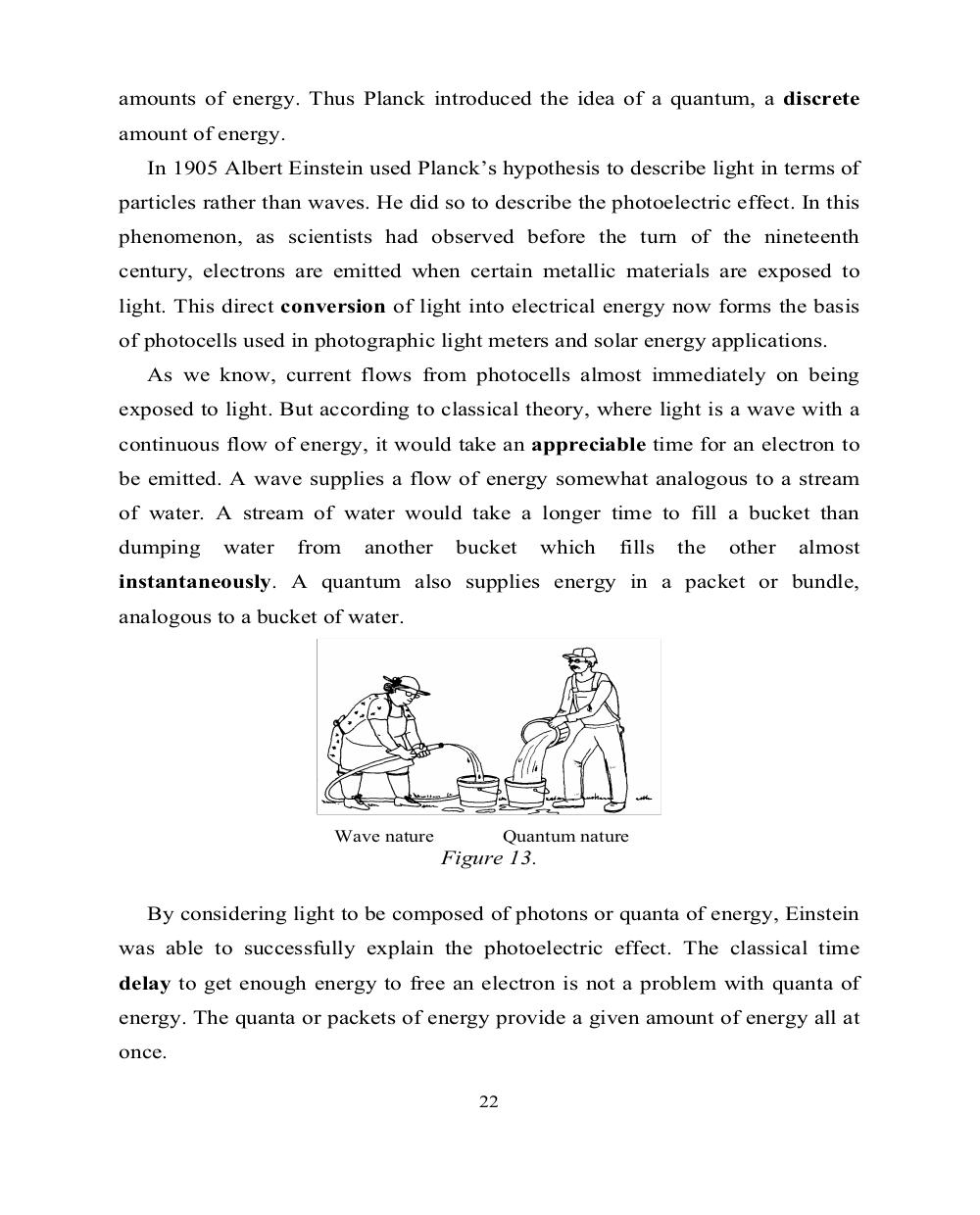# Английский язык. Ч.3 (Units 9,10). Ильичева Н.А - 22 стр.

Составители:

Рубрика:

• ## Иностранный языкamounts of energy. Thus Planck introduced the idea of a quantum, a discrete
amount of energy.
In 1905 Albert Einstein used Planck’s hypothesis to describe light in terms of
particles rather than waves. He did so to describe the photoelectric effect. In this
phenomenon, as scientists had observed before the turn of the nineteenth
century, electrons are emitted when certain metallic materials are exposed to
light. This direct conversion of light into electrical energy now forms the basis
of photocells used in photographic light meters and solar energy applications.
As we know, current flows from photocells almost immediately on being
exposed to light. But according to classical theory, where light is a wave with a
continuous flow of energy, it would take an appreciable time for an electron to
be emitted. A wave supplies a flow of energy somewhat analogous to a stream
of water. A stream of water would take a longer time to fill a bucket than
dumping water from another bucket which fills the other almost
instantaneously. A quantum also supplies energy in a packet or bundle,
analogous to a bucket of water.
Wave nature Quantum nature
Figure 13.
By considering light to be composed of photons or quanta of energy, Einstein
was able to successfully explain the photoelectric effect. The classical time
delay to get enough energy to free an electron is not a problem with quanta of
energy. The quanta or packets of energy provide a given amount of energy all at
once.
22amounts of energy. Thus Planck introduced the idea of a quantum, a discrete
amount of energy.
In 1905 Albert Einstein used Planck’s hypothesis to describe light in terms of
particles rather than waves. He did so to describe the photoelectric effect. In this
phenomenon, as scientists had observed before the turn of the nineteenth
century, electrons are emitted when certain metallic materials are exposed to
light. This direct conversion of light into electrical energy now forms the basis
of photocells used in photographic light meters and solar energy applications.
As we know, current flows from photocells almost immediately on being
exposed to light. But according to classical theory, where light is a wave with a
continuous flow of energy, it would take an appreciable time for an electron to
be emitted. A wave supplies a flow of energy somewhat analogous to a stream
of water. A stream of water would take a longer time to fill a bucket than
dumping    water    from   another     bucket      which   fills   the   other   almost
instantaneously. A quantum also supplies energy in a packet or bundle,
analogous to a bucket of water.

Wave nature           Quantum nature
Figure 13.

By considering light to be composed of photons or quanta of energy, Einstein
was able to successfully explain the photoelectric effect. The classical time
delay to get enough energy to free an electron is not a problem with quanta of
energy. The quanta or packets of energy provide a given amount of energy all at
once.

22